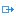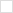Die Dokumentation ab Version 39.5.17 von PLANTA project finden Sie in der neuen PLANTA Online-Hilfe.
TWiki>Current Web>CustomizingDokumentation>PythonIntegration>ServerSeitig>PythonValueRange

# Python Value Ranges

Information
• The following Python methods can be defined in the attribute Wertebereich of all Dataitems.
• They are used to calculate or process the value of a Dataitem.
• You can use the full spectrum of methods available in the Python-API.

## List of Functions

Method Parameter Return value Comment
`computeSqlValueRange(dt_name)` dt_name: String (SQL Alias which PLANTA uses for selecting the current record) The return value of this function is used as a subselect in the original select for this table. So when ever PLANTA selects the current record it embeds the return value of this function to it's initial select as a subquery. Since PLANTA uses aliases as table names, the function receives the currently used table alias as input parameter User defined method is called before the selection process for the current table takes place.
`computeOutput(di)` di: Dataitem Processed new value of the same type as the DI's type User defined method is called for output value computation. If the DI is of string type and length of compute value exceeds DB length of respective DI, the computed value will be trimmed to fit in.
`checkInput(di, oldValue)` di: Dataitem (current DI)

oldValue:same type as the DI's type (old value of DI)

Boolean value that says if check was successful User defined method checks the validity of entered value.
`processInput(di, oldValue)` di: Dataitem (current DI)

oldValue:same type as the DI's type (old value of DI)

Processed new value of the same type as the DI's type User defined method is called during setting of the new value and allow to perform some other action than only simple assigning of the value.

Note: When an incarnation DI has a `processInput()` value range on both sub-DIs only the `processInput()` of the sub-DI with function "ID" is executed when inserting something in this field.

Note:

• PLANTA Server tries to convert the return values of the functions `computeOutput()` and `processInput()` to the underlying DI's type.
• When that fails, it issues a message box containing a Python error similar to the following one:
```Python error in value range of DI041035 "di_customizing_name": computeOutput

<class 'TypeError'>:
argument must be string, not None```

Note:

• Setting datafield or dataitem values using the Python API will never trigger the `checkInput()` function of a Python value range, while `processInput()` will be evaluated.

Information

• The `checkInput()` function is evaluated before `processInput()` is called.
• Thus `checkInput()` will inhibit calling `processInput()` when it does not accept the input.
• `computeOutput()` is evaluated whenever the DI's value is read. No evaluation might happen when PLANTA Server is in echo off mode (using `ppms.echo_off()` or `ppms.echo_disabled()`), as PLANTA Server refreshes the DI-values during the serialization step (which is skipped in echo off mode).

## Dependency Tracking

Information
• Since it is impossible to "auto-detect" all the possible source data references of the respective function, it is necessary to specify them.
• Every function has an dependency attribute, `deps` which contains a tuple of DI source data references. PLANTA then can assure that all references are available when ever this DI is calculated.
• Special feature of the `computeOutput()` function:
• The function is called whenever a source reference changes, this means that the result of the `computeOutput()` function is always up to date according to its source references.

Details

• Following functions must have dependencies:
• `computeOutput()`
• `processInput()`
• `checkInput()`
• The tuple of dependencies can contain
• DI references (DIs from the same record): ‘DI000123'
• Variable references (@G, @D, @L, @M): ‘@G15','@L1'
• '*' meaning that the value has to be recalculated in any case. This will have bad influence on the performance,therefor use this feature with caution.
• It is not necessary to add the DI containing the value range to the dependencies.
• Example: when the `computeOutput()` is customized on DI123456 it's not necessary to add DI123456 to the dependencies.
• If the DI only interacts with itself, simply add `deps = ('',)`
• If there's only one DI in the dependency add `deps = ('DI012345',)`

## Neu ab S 39.5.14

• It is now possible to use a DI's property name (python ID/customizing name) as a dependency.
• When referring to a sibling data item in the same data table, use the plain property name, such as `deps = ('name',)`.
• When referring to a data item from a foreign data table, use that data table's entity name in entity.property notation, such as `deps = ('Module.name',)`.
• Although it is possible to use both DI IDs and Python IDs in the dependencies, PLANTA recommends to only use either the one or the other for uniformity purposes.
• "DI" has to be capitalized, e.g. it has to be `DI000000` instead of `di000000`.

The tuple is specified in the source code of the python value range like this:

```import random
def computeOutput(di):
list = ppms.uvar_get('@M6')
return random.choice(list)
computeOutput.deps = ('@M6',) ```
• In this case, `computeOutput()` depends on @M6 variable.

## Ab S 39.5.14

```def checkInput(di, oldvalue):
rec = di.get_dtp_record()
compl_in_perc=rec.compl_in.get_value() #DI040154
compl_on=rec.compl_on.get_value()      #DI040155
compl_by=rec.compl_by.get_value()      #DI040156

if oldvalue == 0:
if compl_in_perc > 99 and compl_on > 0 and compl_by != "":
return True
else:
return False
else:
return True

# either:
#checkInput.deps = ('DI040154', 'DI040156', 'DI040155', )
# or:
checkInput.deps = ('compl_in', 'compl_on', 'compl_by', )```

## Bis S 39.5.14

```def checkInput(di, oldvalue):
rec = di.get_dtp_record()
compl_in_perc=rec.compl_in.get_value() #DI040154
compl_on=rec.compl_on.get_value()      #DI040155
compl_by=rec.compl_by.get_value()      #DI040156

if oldvalue == 0:
if compl_in_perc > 99 and compl_on > 0 and compl_by != "":
return True
else:
return False
else:
return True

checkInput.deps = ('DI040154', 'DI040156', 'DI040155', )```

### computeOutput

```def computeOutput(di):
rec = di.get_dtp_record()

panel_title = rec.get_di_by_id(57816).get_value()
mod_title = rec.get_di_by_id(1588).get_value()

if panel_title == None or panel_title == '':
return str(mod_title)
else:
return str(panel_title)

computeOutput.deps = ('DI057816', 'DI001588', )```

## Ab S 39.5.14

```def processInput(di, oldvalue):
dtpRec = di.get_dtp_record()
diVal = di.get_value()
name = dtpRec.name.get_value()       #DI001589
dt_id = dtpRec.dt_title.get_value()  #DI003389
if name == "":
dtpRec.name.set_string_value(str(dt_id))
else:
dtpRec.name.set_string_value(str(name))
return str(diVal)

# either:
#processInput.deps = ('DI001589', 'DI003389', )
# or:
processInput.deps = ('name', 'dt_title', )```

## Bis S 39.5.14

```def processInput(di, oldvalue):
dtpRec = di.get_dtp_record()
diVal = di.get_value()
name = dtpRec.name.get_value()       #DI001589
dt_id = dtpRec.dt_title.get_value()  #DI003389
if name == "":
dtpRec.name.set_string_value(str(dt_id))
else:
dtpRec.name.set_string_value(str(name))
return str(diVal)

processInput.deps = ('DI001589', 'DI003389', )```

### computeSqlValueRange

```def computeSqlValueRange(dt_name):
"""Calculate the number of data area assignments"""
val = """
select count(*)
from DT406
where di000969={0}.di000198
""".format(dt_name)

return str(val)```

PLANTA projectSuche in Topic-Namen    Suche in Topic-Inhalten